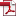## 데이터-재순환 구조에서 적응 가중치 갱신을 통한 LMS 알고리즘 수렴 속 도 개선

Vol. 9, No. 4, pp. 11-22, 8월. 199910.13089/JKIISC.1999.9.4.11, Full Text:Keywords:
Abstract

LMS 적응필터는 많은 신호처리 응용분야에서 광범위하게 사용되고 있으나 반복 적 최소 자승 (RLS) 적응 필터와 비교해서 주어진 안정상태 평균 자승 에러에 대한 수렴특성이 떨어진 다. 본 논문은 LMS 알고리즘의 수렴속도를 향상시키기 위해 폐기된 탭 입력 데이터를 몇 개의 한정된 버퍼를 이용 탭 가중치를 적응적으로 조절하여 수렴특성을 개선한다. 컴퓨터 시뮬레이션 결과를 통해 스텝 크기 매 개변수 $\mu$값의 증가는 보다 빠른 수렴속도와 평균 자승에러를 감소시키는 효과를 가지므로 데이터 재순 환 버퍼 구조에서 탭 가중치의 갱신에 비례하여 평균 자승 에러의 수렴속도가 재순환 버퍼 B를 증가시 켜 수렴속도가 (B+1)배 만큼 증가한다. 데이터 버퍼 알고리즘을 이용한 제안된 TDL 필터가 LMS 알고 리즘과 동일한 수렴조건을 가지고 실행될 때 연산복잡성의 실질적 부담감을 배제하고 채널 간의 상호심 볼간섭을 보다 효율적으로 제어하면서 적응 횡단선 필터의 수렴속도를 증기시켜 개선한다. Least-mean-square(LMS) adaptive filters have proven to be extremely useful in a number of signal processing tasks. However LMS adaptive filter suffer from a slow rate of convergence for a given steady-state mean square error as compared to the behavior of recursive least squares adaptive filter. In this paper an efficient signal interference control technique is introduced to improve the convergence speed of LMS algorithm with tap weighted vectors updating which were controled by reusing data which was abandoned data in the Adaptive transversal filter in the scheme with data recycling buffers. The computer simulation show that the character of convergence and the value of MSE of proposed algorithm are faster and lower than the existing LMS according to increasing the step-size parameter $\mu$ in the experimentally computed. learning curve. Also we find that convergence speed of proposed algorithm is increased by (B+1) time proportional to B which B is the number of recycled data buffer without complexity of computation. Adaptive transversal filter with proposed data recycling buffer algorithm could efficiently reject ISI of channel and increase speed of convergence in avoidance burden of computational complexity in reality when it was experimented having the same condition of LMS algorithm

Statistics